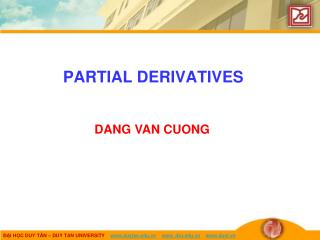DownloadDownload PresentationPARTIAL DERIVATIVES

# PARTIAL DERIVATIVES

Télécharger la présentation## PARTIAL DERIVATIVES

- - - - - - - - - - - - - - - - - - - - - - - - - - - E N D - - - - - - - - - - - - - - - - - - - - - - - - - - -
##### Presentation Transcript

1. PARTIAL DERIVATIVES DANG VAN CUONG

2. PARTIAL DERIVATIVES In general, if f is a function of two variables x and y, suppose we let only x vary while keeping y fixed, say y = b, where b is a constant. • Then, we are really considering a function of a single variable x: g(x) = f(x, b)

3. PARTIAL DERIVATIVE If g has a derivative at a, we call it the partialderivative of f with respect to xat (a, b). We denote it by: fx(a, b) Thus, fx(a,b)=g’(a)where g(x) = f(x, b)

4. PARTIAL DERIVATIVE By the definition of a derivative, we have: So,

5. PARTIAL DERIVATIVE Similarly, the partial derivative of f with respect to yat(a, b), denoted by fy(a, b), is obtained by: • Keeping x fixed (x = a) • Finding the ordinary derivative at bof the function G(y) = f(a, y) Thus,

6. NOTATIONS There are many alternative notations for partial derivatives. • For instance, instead of fx, we can write f1 or D1f (to indicate differentiation with respect to the firstvariable) or ∂f/∂x. • However, here, ∂f/∂x can’t be interpreted as a ratio of differentials.

7. NOTATIONS FOR PARTIAL DERIVATIVES If z = f(x, y), we write:

8. RULE TO FIND PARTIAL DERIVATIVES OF z = f(x, y) Thus, we have this rule. • To find fx, regard y as a constant and differentiate f(x, y) with respect to x. • To find fy, regard x as a constant and differentiate f(x, y) with respect to y.

9. GEOMETRIC INTERPRETATION To give a geometric interpretation of partial derivatives, we recall that the equation z = f(x, y) represents a surface S (the graph of f). • If f(a, b) = c, then the point P(a, b, c) lies on S.

10. GEOMETRIC INTERPRETATION By fixing y = b, we are restricting our attention to the curve C1 in which the vertical plane y = b intersects S. • That is, C1 is the trace of Sin the plane y = b.

11. GEOMETRIC INTERPRETATION Likewise, the vertical plane x = a intersects S in a curve C2. Both the curves C1 and C2 pass through P.

12. GEOMETRIC INTERPRETATION Notice that the curve C1 is the graph of the function g(x) = f(x, b). • So, the slope of its tangent T1at P is: g’(a) = fx(a, b)

13. GEOMETRIC INTERPRETATION The curve C2 is the graph of the functionG(y) = f(a, y). • So, the slope of its tangent T2at P is: G’(b) = fy(a, b)

14. GEOMETRIC INTERPRETATION Thus, the partial derivatives fx(a, b) and fy(a, b) can be interpreted geometrically as: • The slopes of the tangent lines at P(a, b, c) to the traces C1and C2 of S in the planes y = b and x = a.

15. GEOMETRIC INTERPRETATION Example 2 If f(x, y) = 4 – x2 – 2y2 find fx(1, 1) and fy(1, 1) and interpret these numbers as slopes.

16. GEOMETRIC INTERPRETATION Example 2 We have: fx(x, y) = -2xfy(x, y) = -4y fx(1, 1) = -2 fy(1, 1) = -4

17. GEOMETRIC INTERPRETATION Example 2 The graph of f is the paraboloid z = 4 – x2 – 2y2 The vertical plane y = 1 intersects it in the parabola z = 2 – x2, y = 1. • As discussed, we label it C1.

18. GEOMETRIC INTERPRETATION Example 2 The slope of the tangent line to this parabola at the point (1, 1, 1) is: fx(1, 1) = -2

19. GEOMETRIC INTERPRETATION Example 2 Similarly, the curve C2 in which the plane x = 1 intersects the paraboloid is the parabola z = 3 – 2y2, x = 1. • The slope of the tangent line at (1, 1, 1) is: fy(1, 1) = – 4

20. GEOMETRIC INTERPRETATION This is a computer-drawn counterpart to the first figure in Example 2. • The first part shows the plane y = 1 intersecting the surface to form the curve C1. • The second part shows C1 and T1.

21. FUNCTIONS OF MORE THAN TWO VARIABLES Partial derivatives can also be defined for functions of three or more variables. • For example, if f is a function of three variables x, y, and z, then its partial derivative with respect to x is defined as:

22. FUNCTIONS OF MORE THAN TWO VARIABLES It is found by: • Regarding y and z as constants. • Differentiating f(x, y, z) with respect to x.

23. FUNCTIONS OF MORE THAN TWO VARIABLES If w = f(x, y, z), then fx = ∂w/∂x can be interpreted as the rate of change of w with respect to x when y and z are held fixed. • However, we can’t interpret it geometrically since the graph of f lies in four-dimensional space.

24. FUNCTIONS OF MORE THAN TWO VARIABLES In general, if u is a function of n variables,u = f(x1, x2, . . ., xn), its partial derivative with respect to the i th variable xi is:

25. FUNCTIONS OF MORE THAN TWO VARIABLES Then, we also write: